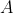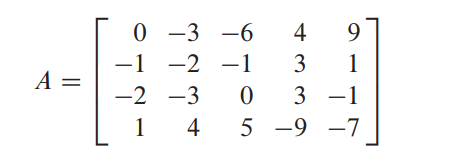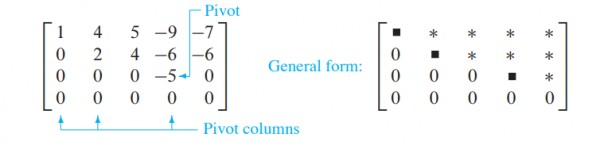# Basis maths-linear algebra-Q2

A  pivot position in a matrixis a location inthat corresponds to a leading 1 in the reduced echelon form of. A pivot column is a column ofthat contains a pivot position.Which of the following is the correct set of Pivot columns of above matrix(Assuming index of first column is 1)

(A).

(B).

(C).

(D).asked May 15
reshown May 16answered May 16 by (112,760 points)